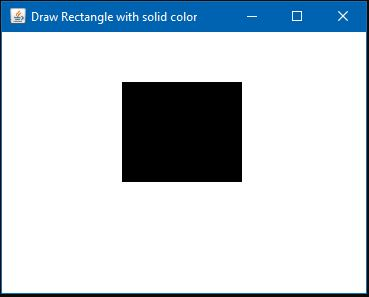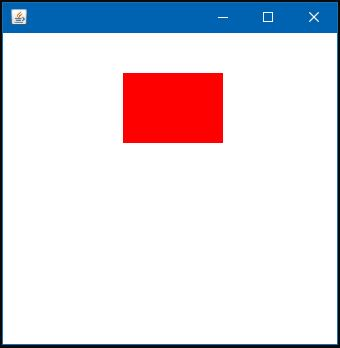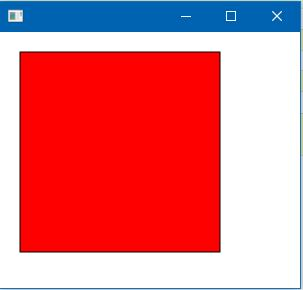# Fill Rectangle in Java

MD Aminul Islam Feb 01, 2023 Sep 01, 2022

You may need to draw rectangles programmatically if you are working with graphics using Java. This article will show how we can draw a rectangle in Java and fill it with color.

## Use the `fillRect()` and `paint()` Method to Fill a Rectangle in Java Applet

In our example below, we will draw and fill a rectangle using the `Java Applet`.

Code Example

``````import java.applet.Applet;
import java.awt.*;
import java.awt.event.*;
public class DrawRect extends Applet
{
public static void main(String[] args)
{
Frame DrawRectangle = new Frame("Draw Rectangle with solid color");
DrawRectangle.setSize(380, 300);
Applet DrawRect = new DrawRect();
DrawRectangle.setVisible(true);
public void windowClosing(WindowEvent e) {System.exit(0); }

});
}
public void paint(Graphics Grph)
{
Grph.fillRect(120, 50, 120, 100);
}
}
``````

In the example above, we created a frame and set its size. After that, we draw a rectangle using the `Applet DrawRect = new DrawRect();` and then add it to the frame.

Then, we make the frame visible. Here the `paint()` method is used to colorize the rectangle.

Output:## Use the `fillRect()` and `paint()` Method to Fill a Rectangle in Java Swing

In our example below, we will draw and fill a rectangle using the Java Swing.

Code Example:

``````import java.awt.*;
import javax.swing.JFrame;

public class SwingRect extends Canvas{

public void paint(Graphics Grph) {
setBackground(Color.WHITE);
Grph.fillRect(120, 40, 100, 70);
setForeground(Color.RED);
}
public static void main(String[] args) {
SwingRect rect = new SwingRect();
JFrame frm = new JFrame();
frm.setDefaultCloseOperation(JFrame.EXIT_ON_CLOSE);
frm.setSize(350,350);
frm.setVisible(true);
}
}
``````

In the example above, we create a rectangle object using the line `SwingRect rect = new SwingRect();`.

Output:## Use the `setFill()` Method to Fill Rectangle in JavaFX

In our example below, we will draw and fill a rectangle using JavaFX.

Code Example:

``````import javafx.application.Application;
import javafx.scene.Group;
import javafx.scene.Scene;
import javafx.scene.paint.Color;
import javafx.scene.shape.Rectangle;
import javafx.stage.Stage;

public class JavaFxRect extends Application {

public static void main(String[] args) {
Application.launch(args);
}

@Override
public void start(Stage MyStage) {
Group MyGroup = new Group();
Rectangle MyRect = new Rectangle(20, 20, 200, 200);
MyRect.setFill(Color.RED);
MyRect.setStroke(Color.BLACK);
Scene MyScene = new Scene(MyGroup, 300, 200);
MyStage.setScene(MyScene);
MyStage.show();
}
}
``````

In the example above, we created a group object, then created a rectangle object using the line `Rectangle MyRect = new Rectangle(20, 20, 200, 200);`. Now we filled the rectangle object with red color by using the line `MyRect.setFill(Color.RED);`.

Also, we set a stroke to the rectangle by the line `MyRect.setStroke(Color.BLACK);`. After that, we add the rectangle to the group. We created a scene and included it on stage, and made the stage visible.

Output:Aminul Is an Expert Technical Writer and Full-Stack Developer. He has hands-on working experience on numerous Developer Platforms and SAAS startups. He is highly skilled in numerous Programming languages and Frameworks. He can write professional technical articles like Reviews, Programming, Documentation, SOP, User manual, Whitepaper, etc.